## Customizable defaults for Positioning Functions

Normally sensible default label positions are chosen for you automatically when you call direct.label(p) with a plot p. But what if you don't like the defaults? For individual plots you can always specify a particular Positioning Function f by using the second argument: direct.label(p,f).

But what if you consistently think that the default choice is bad, and you would like to redefine it once and for all? You can define a custom function that chooses the default direct label positions, in the following manner. Put the following code in your ~/.Rprofile, and edit it to reflect your desired defaults for lattice and/or ggplot2 plots:

```options(
directlabels.defaultpf.trellis=function(lattice.fun.name,groups,type,...){
ldefault <- if(nlevels(groups)==2)"lines2" else "maxvar.points"
switch(lattice.fun.name,
dotplot=ldefault,
xyplot=switch(type,p="smart.grid",ldefault),
densityplot="top.points",
qqmath=ldefault,
rug="rug.mean",
stop("No default direct label placement method for '",
},
directlabels.defaultpf.ggplot=function(geom,...){
switch(geom,
density="top.points",
line="maxvar.points",
point="empty.grid.2",
path="bottom.points",
stop("No default label placement for this type of ggplot."))
})
```

## Labeling several positions

What if it makes sense to put a group label at more than one place for increased clarity? Then all you have to do is construct a Positioning Function that returns several rows for each group.

A special case: if you want to combine the output from several existing Positioning Functions, there is a shortcut function that can help! dl.combine is included in the directlabels package, and it works like this:

```data(BodyWeight,package="nlme")
library(lattice)
P <- xyplot(weight~Time|Diet,BodyWeight,groups=Rat,type="l",layout=c(3,1))
library(directlabels)
direct.label(P,dl.combine(first.points,last.qp))
```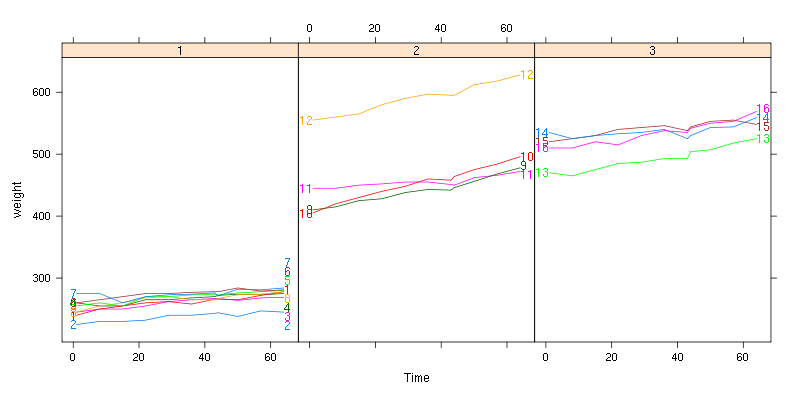## Labeling black and white plots for printout

 ```library(lattice) trellis.par.set(standard.theme(color=FALSE)) p <- xyplot(jitter(Sepal.Length)~jitter(Petal.Length),iris,groups=Species) direct.label(p) ```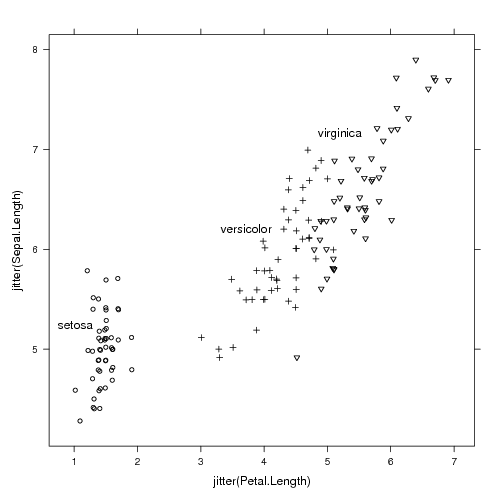```library(ggplot2) ggplot(iris,aes(Petal.Length,Sepal.Length))+ geom_point(aes(shape=Species))+ geom_dl(aes(label=Species),method="smart.grid")+ scale_shape_manual(values=c(setosa=1,virginica=6,versicolor=3),guide="none") ```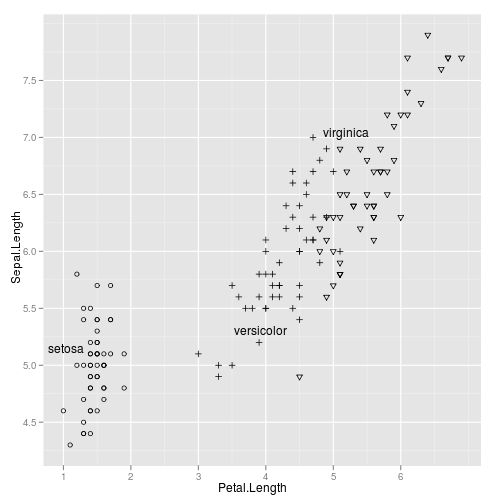## Custom panel and panel.groups functions for the rat body weight data

If you want to use a custom display in lattice panels, you need to write a custom panel or panel.groups function. For something you want to draw once for each panel, use a custom panel function, and for something that you want to draw for each group, use a custom panel.groups function. When you use a custom panel.groups function, you need to explicitly specify the Positioning Function for the direct labels. This series of examples should should illustrate how to effectively create custom direct labeled displays.

```library(directlabels)
data(BodyWeight,package="nlme")
## Say we want to use a simple linear model to explain rat body weight:
fit <- lm(weight~Time+Diet+Rat,BodyWeight)
bw <- fortify(fit,BodyWeight)

## Custom panel function which highlights min and max values:
panel.range <- function(y,...){
panel.abline(h=range(y))
panel.superpose(y=y,...)
}
direct.label(xyplot(weight~Time|Diet,bw,groups=Rat,type="l",
layout=c(3,1),panel=panel.range))
```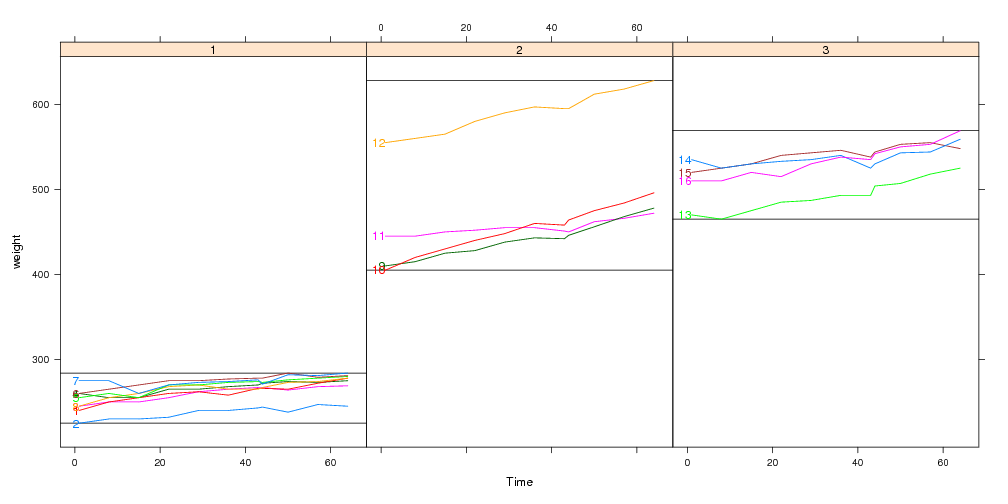```## This panel.groups function will display the model fits:
panel.model <- function(x,subscripts,col.line,...){
panel.xyplot(x=x,subscripts=subscripts,col.line=col.line,...)
llines(x,bw[subscripts,".fitted"],col=col.line,lty=2)
}
x <- xyplot(weight~Time|Diet,bw,groups=Rat,type="l",layout=c(3,1),
panel=panel.superpose,panel.groups=panel.model)
direct.label(x,"first.points")
```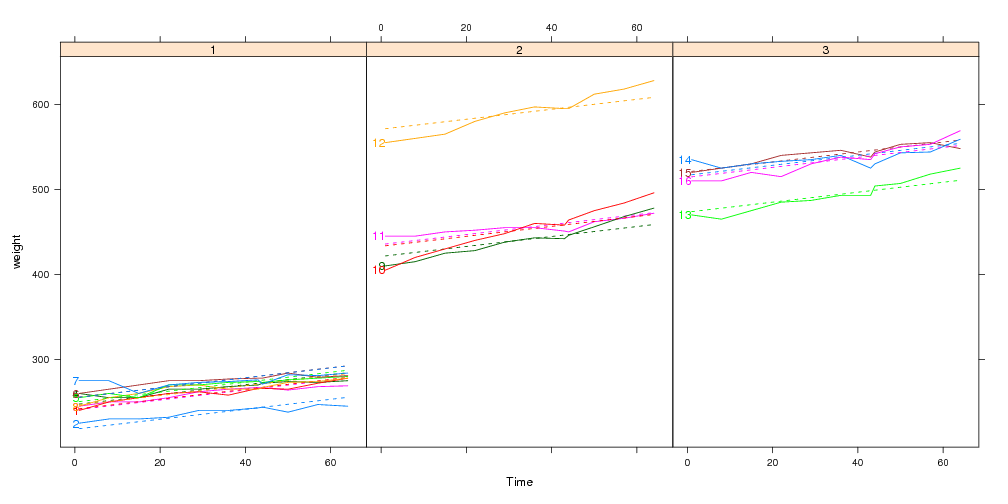```## Custom panel and panel.groups functions:
x <- xyplot(weight~Time|Diet,bw,groups=Rat,type="l",layout=c(3,1),
panel=panel.range,panel.groups=panel.model)
direct.label(x,"first.points")
```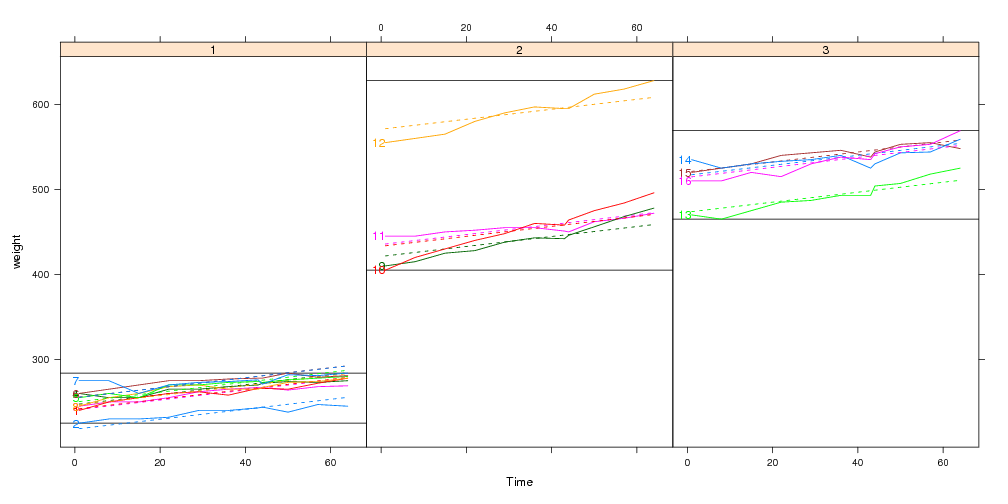## Using the panel.superpose.dl panel function

The panel.superpose.dl function can be used in place of panel.superpose in your lattice plots. It behaves just like panel.superpose, but it intelligently adds direct labels. If you specify panel.groups as a character rather than a function, then we can guess a Positioning Function. If not, you can always specify a Positioning Function with the method= argument.

```xyplot(weight~Time|Diet,bw,groups=Rat,type="l",layout=c(3,1),
panel=panel.superpose.dl,panel.groups="panel.xyplot")
```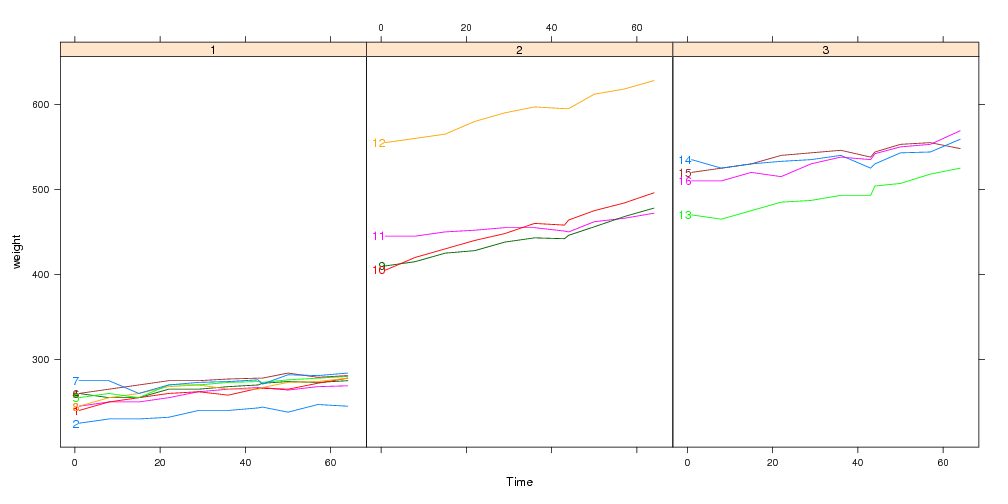Here we use a custom panel.groups function so the Positioning Function must be specified using the method= argument:

```xyplot(weight~Time|Diet,bw,groups=Rat,type="l",layout=c(3,1),
panel=panel.superpose.dl,panel.groups=panel.model,method="first.points")
```## Specifying the positioning method as a list

Since the input and output of a Positioning Function is the same sort of data frame, you can chain Positioning Functions together. To illustrate how this works, consider the following contrived example:

```complicated <- list(dl.trans(x=x+1), ## shift every point to the right 1cm
gapply.fun(d[-2,]), ## delete the 2nd point of every group
rot=c(30,180)) ## rotate by alternately 30 and 180 degrees
```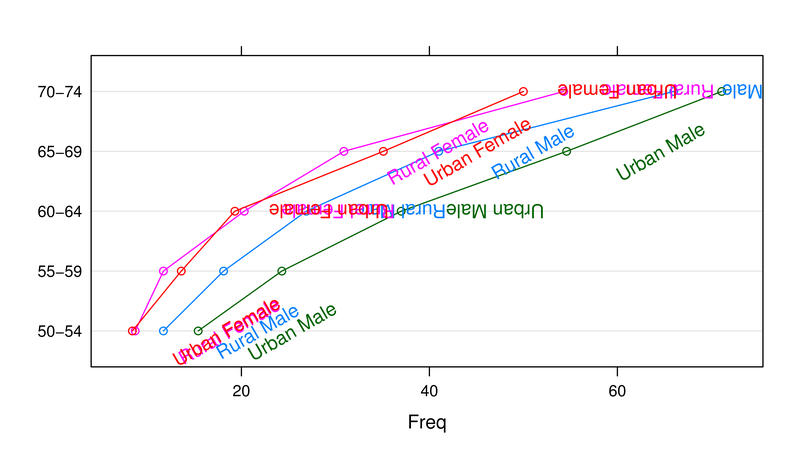When method is specified as a list, the arguments will be applied in sequence, starting from the data frame of all plotted points. In this example,

1. In Positioning Methods the x and y units are centimeters on the plotting device. The first list element dl.trans(x=x+1) is a function that adds 1 to every x value, and has the effect of shifting the labels to the right 1cm.
2. The second element is a call to gapply.fun, which lets you specify an expression to apply to each group, as a function of the data d. In this case d[-2,] means delete the second row from each group.
3. Named elements are copied to the data frame, so the third element, rot=c(30,180), adds a rot column to the data.frame, with values 30 and 180 for every other row. This has the effect of rotating every label by 30 or 180 degrees. Text display parameters alpha, fontsize, fontfamily, fontface, lineheight, and cex can also be specified in this manner (see the help pages for grid::gpar for an exhaustive description and directlabels::dlcompare for an example).

## Compare several plots and methods

Several different labeling methods can be compared for any mix of ggplot2 and/or lattice plots.

```library(directlabels)
library(reshape2)
dts <- cbind(male=mdeaths,female=fdeaths,time=1:length(mdeaths))
ddf <- melt(as.data.frame(dts),id="time")
names(ddf) <- c("time","sex","deaths")
plots <- list(lattice=
xyplot(deaths~time,ddf,groups=sex,type="l",xlim=c(-15,80)),
ggplot2=
qplot(time,deaths,data=ddf,colour=sex,geom="line")+xlim(-10,80))
dlcompare(plots,list("first.points","lines2"))
```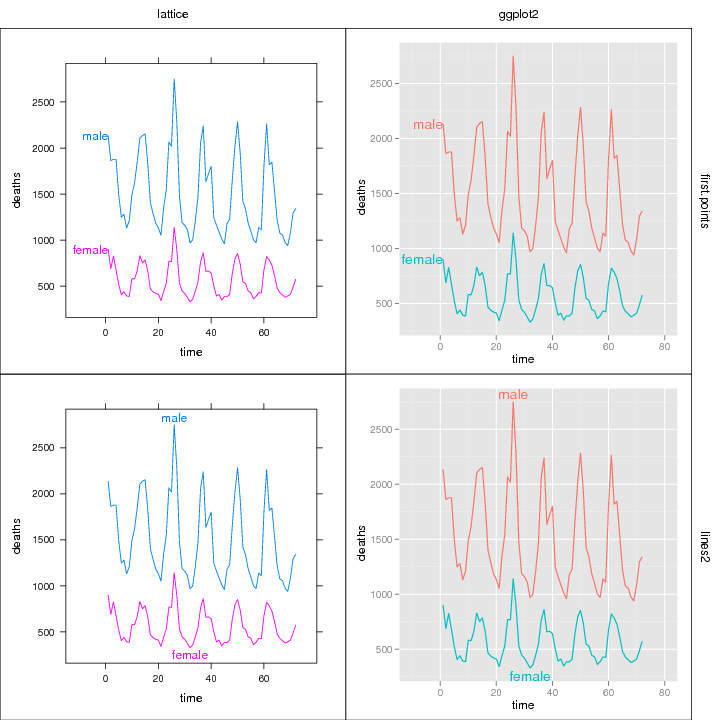Please send email to Toby Dylan Hocking if you are using directlabels or have ideas to contribute, thanks! validate